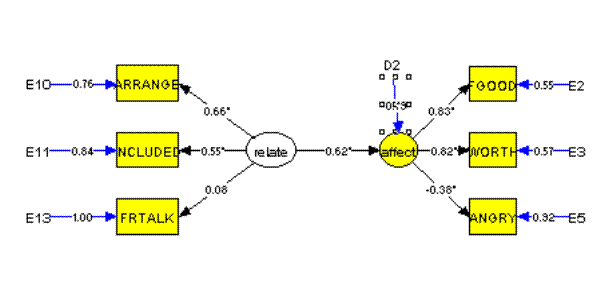EQS Rules, Definitions and Operations

Merle Canfield

The block diagrams can be used first to create a model or theory.  That diagram can then be used to create the equations for testing the model.  The block diagram then represents the structural model to be tested.  The following are rules for drawing the block diagrams.

1.            Variables in the input data file to be analyzed are observed or measured variables; called Vs in EQS (p 13).  They are represented by rectangles in the block diagram.

2.            Hypothetical constructs are unmeasured latent variables, as in factor analysis, and are called factors or Fs (p 13).  They are represented by circles in the block diagram.

3.            Residual variation in measured variables is generated by errors or Es (p 13).  Represented by E on the block diagram.

4.            Residual variation of unmeasured (latent) variables is generated by errors in the equations are called Ds or disturbances in EQS (p 18).  Represented by D on the block diagram.

5.            A straight arrow with a single pointer represents casual or directional influence of one variable (either measured or latent) on another (p. 17).

6.            An arrow with dual pointers (the arrow can be either curved or straight) represents a correlation or covariation between two variables (it is sometimes not curved -- the dual pointers determine it's nature) (p. 17).  These represent regression coefficients.

7.            All arrows represent parameters of the equation.

8.            Arrows that have asterisks indicate that the parameter is to be estimated.

9.            Arrows that have a "1" indicate that the parameter is set to be 1.

10.          Arrows that have a "0" indicate that the parameter is set to be 0.

11.          Arrows that have no symbols indicate that the parameter is set to be 1.

12.          An absence of arrows between two elements (error or variables) indicates that the path is set to 0.

13.          EQS cannot deal with dependent categorical variables (p 5).

14.          The ratio of sample size to number of free parameters to be estimated may be able to go as low as 5:1 under normal and elliptical theory (p 6).

15.          There is one equation for each dependent variable in the system (p 7).

16.          A dependent variable is one that is a structured regression function of other variables; it is recognized in a path diagram by having one or more arrows aiming at it (p 7).

17.          Every independent variable in the model must have a variance (p 7).

18.          Dependent variables cannot have variances or covariances with other variables as parameters of a structural model (p 16).

19.          df = measured variables * (measured variables + 1)/2 (p 18).

20.          Every unmeasured variable (i.e. F, E, and D variables) in a structural model must have its scale determined.  This can always be done by fixing a path from that variable to another variable at some known value (usually 1.0).  An alternative method for determining the scale of an independent unmeasured variable is to fix its variance at some known value (usually 1.0) (p 18).

21.          When the V999 variable is used a /MEANS command is required (p. 168).

22.          When using the error variance of a variable or a factor to assess the variance accounted for and test such variance with a chi square change by removing an arrow this can be done only with an arrow pointing at the variable being assessed.  The arrow removed must be the only change in the model.

23.          Except for special cases a factor must have at least two variables.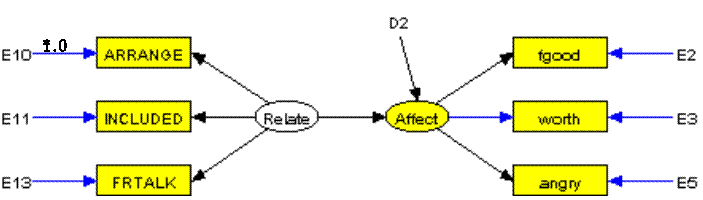RUNNING EQS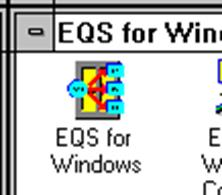Double click on the "EQS for Windows" icon and the following window appears: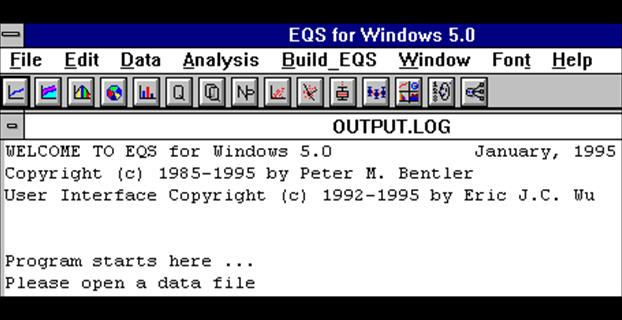Click on File and the next window appears: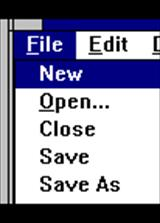Click on Open and the next window appears: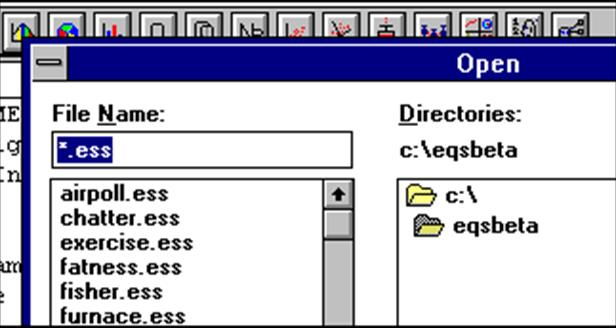Type "\students\lsqrnd2.ess" in the File Name box so that it looks like this: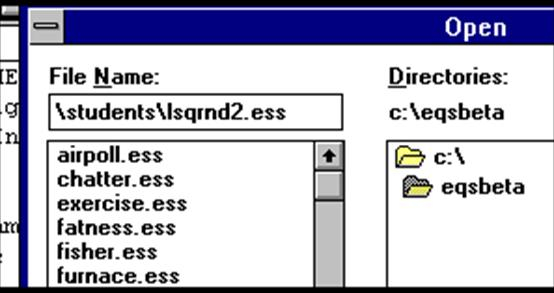Click OK or press return and the following window appears: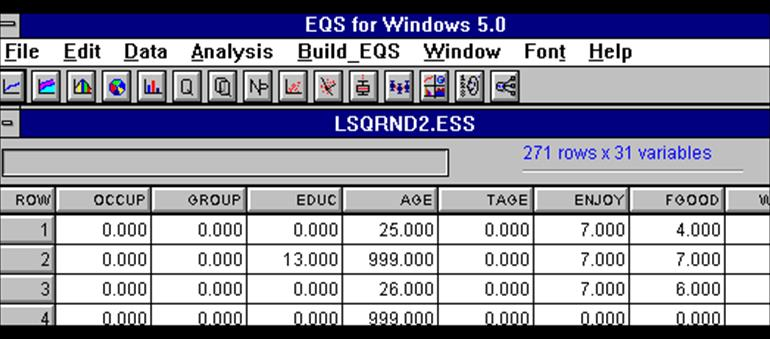Click on the last button of the "button bar" or "power bar" (it is just below the last 'w' of Window) and the next screen will appear: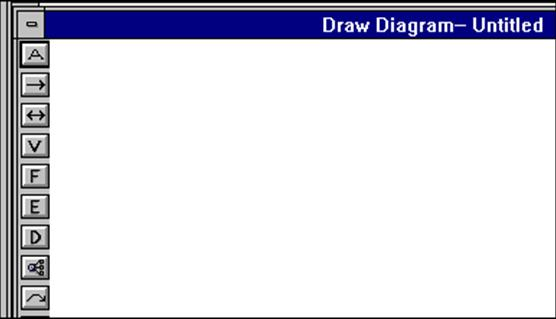Click on the button below the "D" (it is similar to the previous button selected).  Move the + to the left side of the "drawing area."  The following window will appear: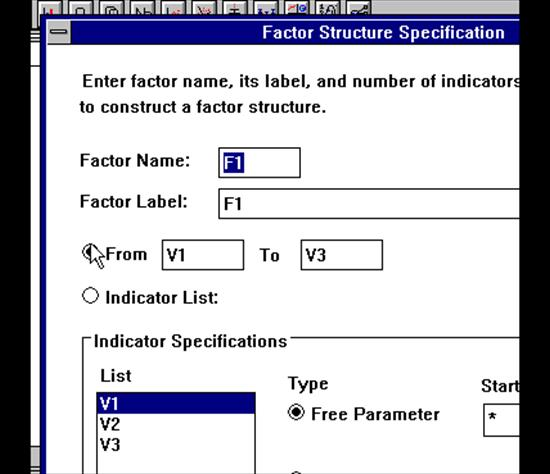It should be filled in like the next box: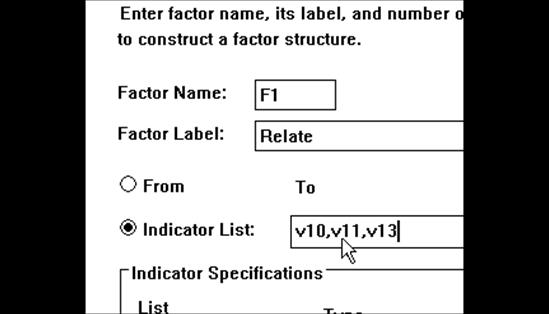And then click Apply; click OK.  The following graph will appear: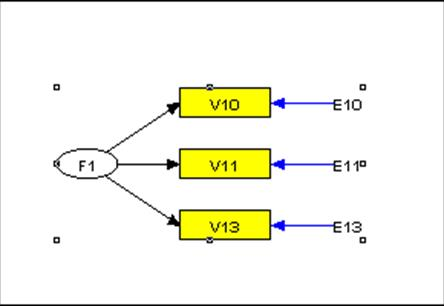It is turned the wrong direction.

Click Edit;click Select All

Click Edit; click Horizontal Flip

Click the "cat paw" again (the button just below the "D"):

Move the + to the right side of the drawing area and click:

File in the window like this: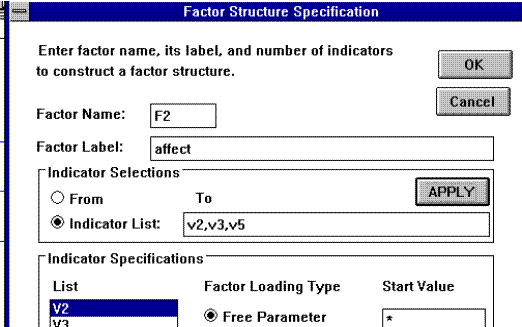Click Apply;

click OK

Click Edit; click Select All

Click Edit; click Align Horizontal

Click on "right arrow" button: move + to just inside the right side of the F1 circle and click

move + to just inside the left side of the F2 circle and click

Click View

Click Labels

The drawing should look like this: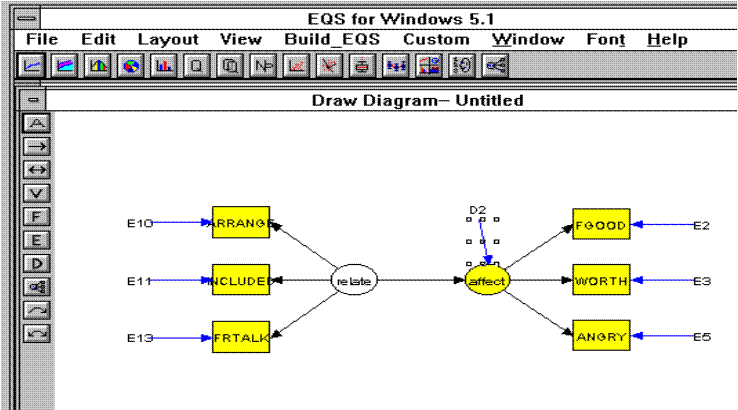Click File

Click Save As

File in the window as follows: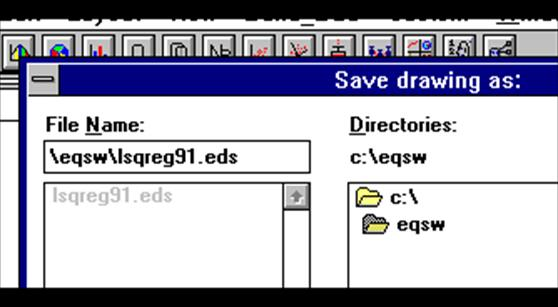Click OK; click "Yes"

Click Build_EQS; click Title/Specifications

File in the window as follows: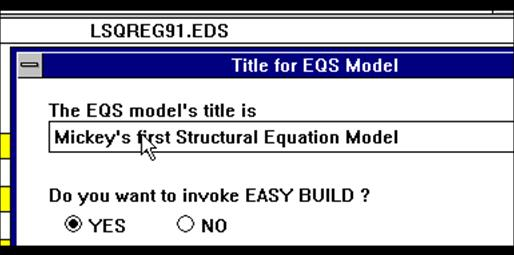Click OK

Click OK to the next window and the following jobstream appears: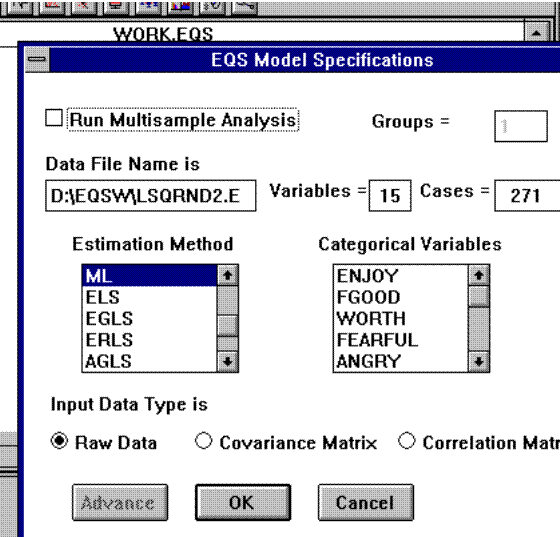save it

Click on Build_EQS

Click on Run EQS/386

Click OK

Save File

Select Diagram

Click View

Select Estimates

Select Standardized Solution

The diagram should look like this.